Exit Ticket for Day 37 of Calculus
Please select your name from the following list: *
Here is the "horrible" equation for the first problem: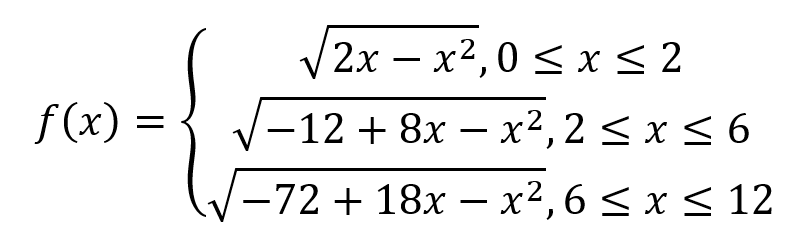Here is what a graph of that horrible equation looks like: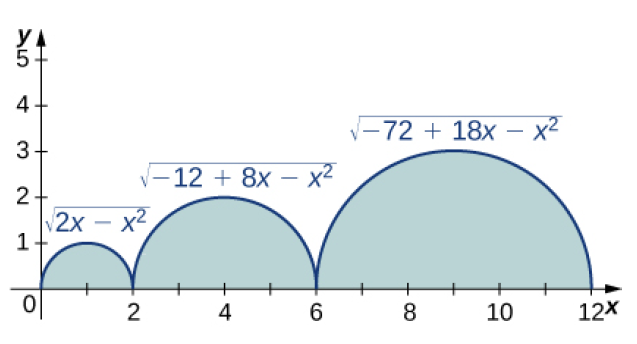1. For the horrible equation (and graph) above, evaluate the following: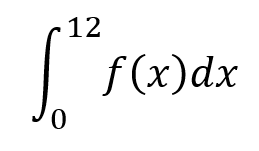2. Review question: What is the antiderivative of this expression?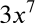3. Review question: Find the derivative of this function: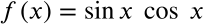4. REVIEW QUESTION: The function shown below has a critical point at x=1.470628906. (That happens to be the inverse cosine of 0.1). Is that critical points associated with a relative MAXIMUM or a relative MINIMUM of the function?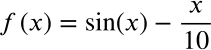This form was created inside of Evansville Vanderburgh School Corporation. Report Abuse - Terms of Service